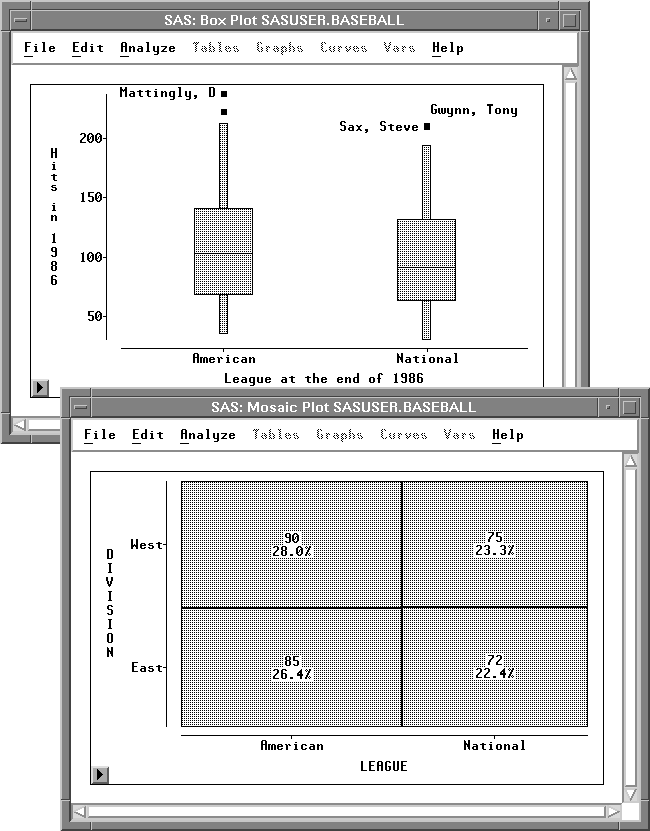Previous Page Next Page
 Box Plots and Mosaic Plots

# Box Plots and Mosaic Plots

Box plots are pictorial representations of the distribution of values of a variable. The central line in each box marks the median value and the edges of the box mark the first and third quartiles.

The median value of a distribution is the 50th percentile: It is the value less than and greater than 50% of the data. The first and third quartiles are the 25th and 75th percentiles. By combining these three values in a schematic diagram and plotting individual markers for extreme data values, the box plot provides a concise display of a distribution (Tukey 1977).

Mosaic plots are pictorial representations of frequency counts of a single nominal variable or cross-classified nominal variables. Because mosaic plots display the frequencies graphically, they are easier to understand than crosstabulations. You can select and brush mosaic plots to explore dependencies between variables.Figure 33.1: Box Plot and Mosaic Plot

#### References

 Previous Page Next Page Top of Page